Olympiad Test Level 1: Numbers - 2

# Olympiad Test Level 1: Numbers - 2

Test Description

## 15 Questions MCQ Test Math Olympiad for Class 2 | Olympiad Test Level 1: Numbers - 2

Olympiad Test Level 1: Numbers - 2 for Class 2 2022 is part of Math Olympiad for Class 2 preparation. The Olympiad Test Level 1: Numbers - 2 questions and answers have been prepared according to the Class 2 exam syllabus.The Olympiad Test Level 1: Numbers - 2 MCQs are made for Class 2 2022 Exam. Find important definitions, questions, notes, meanings, examples, exercises, MCQs and online tests for Olympiad Test Level 1: Numbers - 2 below.
Solutions of Olympiad Test Level 1: Numbers - 2 questions in English are available as part of our Math Olympiad for Class 2 for Class 2 & Olympiad Test Level 1: Numbers - 2 solutions in Hindi for Math Olympiad for Class 2 course. Download more important topics, notes, lectures and mock test series for Class 2 Exam by signing up for free. Attempt Olympiad Test Level 1: Numbers - 2 | 15 questions in 30 minutes | Mock test for Class 2 preparation | Free important questions MCQ to study Math Olympiad for Class 2 for Class 2 Exam | Download free PDF with solutions
 1 Crore+ students have signed up on EduRev. Have you?
Olympiad Test Level 1: Numbers - 2 - Question 1

### Which option is same as one hundred?

Olympiad Test Level 1: Numbers - 2 - Question 2

### If 941 = 900 + a + 1, then a =

Detailed Solution for Olympiad Test Level 1: Numbers - 2 - Question 2

941 = 900 + a + 1
941 = 901 + a
a = 941 – 901
a = 40

Olympiad Test Level 1: Numbers - 2 - Question 3

### Select the correct match.

Detailed Solution for Olympiad Test Level 1: Numbers - 2 - Question 3

70 tens – 5 tens = 65 tens = 650 = six hundred and fifty

Olympiad Test Level 1: Numbers - 2 - Question 4

Three hundred and one can be written as _________ .

Olympiad Test Level 1: Numbers - 2 - Question 5

What is the place value of the digit 7 in 5001725?

Detailed Solution for Olympiad Test Level 1: Numbers - 2 - Question 5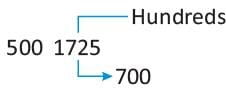Olympiad Test Level 1: Numbers - 2 - Question 6

Which one of the following is correct for 543?

Detailed Solution for Olympiad Test Level 1: Numbers - 2 - Question 6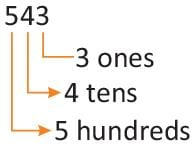Olympiad Test Level 1: Numbers - 2 - Question 7

Which one of the following is correct about the places of digits 4, 5, 6 and 7 in the number 7654?

Detailed Solution for Olympiad Test Level 1: Numbers - 2 - Question 7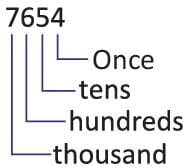Olympiad Test Level 1: Numbers - 2 - Question 8

What is the nearest hundred for 186?

Detailed Solution for Olympiad Test Level 1: Numbers - 2 - Question 8

As 8 is in tens place, so nearest hundred for 186 is 200.

Olympiad Test Level 1: Numbers - 2 - Question 9

4 hundreds + 2 tens is same as:

Detailed Solution for Olympiad Test Level 1: Numbers - 2 - Question 9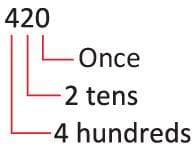Olympiad Test Level 1: Numbers - 2 - Question 10

What is the common digit at the place of ones in numbers 27, 37, 57 and 87?

Detailed Solution for Olympiad Test Level 1: Numbers - 2 - Question 10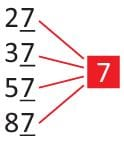Olympiad Test Level 1: Numbers - 2 - Question 11

Find the nearest number for following question:

Nearest tens for 96

Detailed Solution for Olympiad Test Level 1: Numbers - 2 - Question 11

As 6 is in ones place, so 100 is the nearest tens.

Olympiad Test Level 1: Numbers - 2 - Question 12

Find the nearest number for following question:

Nearest hundred for 202

Detailed Solution for Olympiad Test Level 1: Numbers - 2 - Question 12

As 0 is in tens place, so 200 is the nearest hundred.

Olympiad Test Level 1: Numbers - 2 - Question 13

Find the nearest number for following question:

Nearest hundred for 176

Detailed Solution for Olympiad Test Level 1: Numbers - 2 - Question 13

As 7 is in tens place, so 200 is the nearest hundred.

Olympiad Test Level 1: Numbers - 2 - Question 14

Find the nearest number for following question:

Nearest tens for 43

Detailed Solution for Olympiad Test Level 1: Numbers - 2 - Question 14

As 3 is in ones place, so 40 is the nearest tens.

Olympiad Test Level 1: Numbers - 2 - Question 15

Which of the following is true about the number 234?

Detailed Solution for Olympiad Test Level 1: Numbers - 2 - Question 15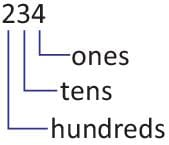## Math Olympiad for Class 2

72 tests
 Use Code STAYHOME200 and get INR 200 additional OFF Use Coupon Code
Information about Olympiad Test Level 1: Numbers - 2 Page
In this test you can find the Exam questions for Olympiad Test Level 1: Numbers - 2 solved & explained in the simplest way possible. Besides giving Questions and answers for Olympiad Test Level 1: Numbers - 2, EduRev gives you an ample number of Online tests for practice

72 tests